Worksheet Solution: Data Handling

# Worksheet Solution: Data Handling - Mathematics (Maths) Class 7

Q.1. Find the mode of the following data:
24, 26, 23, 26, 22, 25, 26, 28

Ans: Arranging the given data with the same value together, we get
22, 23, 24, 25, 26, 26, 26, 28
Here, 26 occurs the greatest number of times i.e. 3 times
Thus, the required mode = 26.

Q.2. Find the median of the following data:
8, 6, 10, 12, 14

Ans: Let us arrange the given data in increasing order,
6, 8, 10, 12, 14
n = 5 (odd)
Median = (n + 1/2)th term = 3rd term = 10
Thus, the required median = 10.

Q.3. A fair die is rolled, find the probability of getting a prime number.
Ans: Number on a die = 1, 2, 3, 4, 5, 6
n(S) = 6
Prime numbers = 2, 3, 5
n(E) = 3
Probability = n(E) / n(S) = 3 / 6 = 1 / 2
Thus the required probability = 1 / 2.

Q.4. Find the mean of the first 5 multiples of 3.
Ans: Five multiples of 3 are 3, 6, 9, 12 and 15
Mean =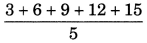= 45 / 5
= 9

Q.5. Find the mean and median of first five prime numbers.
Ans: First five prime numbers are: 2, 3, 5, 7 and 11
Mean =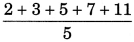= 28 / 5
= 5.6

Q.6. A bag contains 5 white and 9 red balls. One ball is drawn at random from the bag. Find the probability of getting
(a) a white ball
(b) a red ball

Ans: Total number of balls = 5 + 9 = 14 balls
(a) Number of white ball = 5
n(E) = 5
Probability of getting white ball = n(E)/n(S) = 5 / 14
(b) Number of red balls = 9
n(E) = 9
Probability of getting white ball = n(E)/n(S) = 9 / 14

Q.7. Find the range of the following data:
21, 16, 30, 15, 16, 18, 10, 24, 26, 20

Ans: Greatest number 30
Smallest number = 10
Range = 30 – 10 = 20

Q.8. Find the average of the numbers 8, 13, 15.
Ans: Average = Sum of the numbers / Total number of terms
= 8 + 13 + 15 / 3
= 36/3
= 12

Q.9. Find the median of the following data:
20, 14, 6, 25, 18, 13, 19, 10, 9, 12

Ans: Arranging the given data in increasing order, we get
6, 9, 10, 12, 13, 14, 18, 19, 20, 25
n = 10 (even)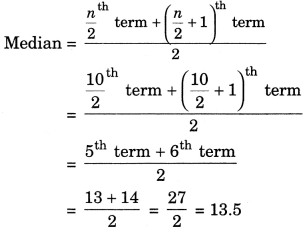Thus, the required median = 13.5

Q.10. The following bar graph shows the number of books sold by a publisher during the five consecutive years. Read the bar graph and answer the following questions:
(i) About how many books were sold in 2008, 2009 and 2012 years?
(ii) In which years were 575 books were sold?
(iii) In which years were the minimum number of books sold?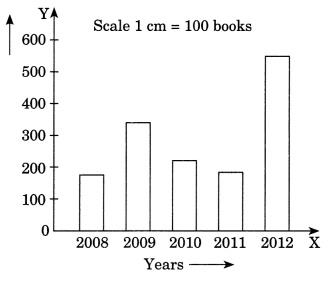Ans:
(i)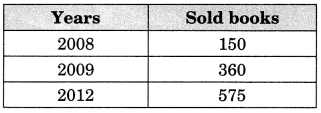(ii) In the year of 2012, maximum number of books i.e. 575 were sold.
(iii) Minimum number of books i.e. 150 were sold in the year 2008.

Q.11. The marks obtained (out of 10) by 80 students in a class test are given below: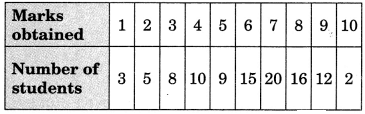Find the mode of the above data.

Ans: In the given frequency distribution table, we find that the observation 7 has maximum frequency, i.e., 20
Hence, the required mode = 7.

Q.12. State true of false.
(i) A data set may have more than one mode.
(ii) The data set 4, 8, 11, 7, 5, 0 has the mode 0.
(iii) The median can be value which is not equal to any of the observation.
(iv) It is impossible to get a sum of 14 of the numbers on both dice when a pair of dice is thrown together
(v) Mean of the observations can be lesser than each of the observations.
(vi) Mean of a data set is the value which divides the value into two equal parts.
(vii) The value of median changes if the value in a data set are arranged in ascending order instead of descending order.
(viii) Rolling the number 7 on a standard die is an impossible event.
(ix) Mean of the data is always from the given data.
(x) Mode of the data is always from the given data.

Ans: (i) - True
(ii) - False
(iii) - False
(iv) - True
(v) - False
(vi) - False
(vii) - False
(viii) - True
(ix) - False
(x) - True

Q.13. A batsman scored the following number of runs in six innings:
36, 35, 50, 46, 60, 55
Calculate the mean runs scored by him in an inning

Ans: 47

Q.14. Fill in the blanks:
(i) The difference between the highest and lowest values of observations in a set of data is called the_________
(ii) The representation of numbers using bars of uniform width, the length of the bars depicting the frequency is called the_________
(iii) When the raw data is arranged in ascending or descending order, it forms an_________
(vi) The probability of getting 1 in tossing of coin is_________
(v) The outcomes which have equal chances of occurrence are called_________.
(vi) Different forms of central tendency are _________and__________
(vii) On dividing the sum of observation by total number of observations, we obtain the_________
(viii) The possible results of an experiment are called_________
(ix) The measure of chance of happening something is called_________
(x) The probability of getting a tail in a throw of a dice is_________.

Ans: (i) - Range
(ii) - Bar graph
(iii) - array
(iv) - 0
(v) - equally likely
(vi) -  Mean, median and Mode
(vii) - mean
(viii) - outcomes
(ix) - probability
(x) - 0

Q.15. The mean of 5 numbers is 25. If the four numbers 16, 26, 31, 32. Find the fifth numbers.
Ans: 20

The document Worksheet Solution: Data Handling | Mathematics (Maths) Class 7 is a part of the Class 7 Course Mathematics (Maths) Class 7.
All you need of Class 7 at this link: Class 7

## Mathematics (Maths) Class 7

121 videos|351 docs|45 tests

## FAQs on Worksheet Solution: Data Handling - Mathematics (Maths) Class 7

 1. What is data handling in class 7?Ans. Data handling in class 7 refers to the process of collecting, organizing, analyzing, and interpreting data. It involves various techniques such as creating tables, graphs, and charts to represent data in a meaningful way.
 2. How can I collect data for a data handling project in class 7?Ans. There are several ways to collect data for a data handling project in class 7. You can conduct surveys, interviews, or observations to gather information. You can also use existing data sources such as books, websites, or databases to collect relevant data.
 3. What are the different methods of organizing data in class 7?Ans. In class 7, data can be organized using various methods such as creating frequency tables, tally marks, or bar graphs. You can also use pictographs or line graphs to represent data. The choice of method depends on the type of data and the purpose of the analysis.
 4. How can I analyze data in class 7?Ans. Data analysis in class 7 involves examining the collected data to identify patterns, trends, or relationships. You can calculate measures of central tendency such as mean, median, or mode to summarize the data. You can also use measures of dispersion such as range or standard deviation to understand the spread of the data.
 5. Why is data handling important for class 7 students?Ans. Data handling is important for class 7 students as it helps develop critical thinking and analytical skills. It enables students to make sense of information, draw conclusions, and make informed decisions. Data handling also enhances their mathematical and statistical abilities, which are essential in various real-life situations.

## Mathematics (Maths) Class 7

121 videos|351 docs|45 testsExplore Courses for Class 7 examSignup to see your scores go up within 7 days! Learn & Practice with 1000+ FREE Notes, Videos & Tests.
10M+ students study on EduRev
Track your progress, build streaks, highlight & save important lessons and more!
Related Searches

,

,

,

,

,

,

,

,

,

,

,

,

,

,

,

,

,

,

,

,

,

;